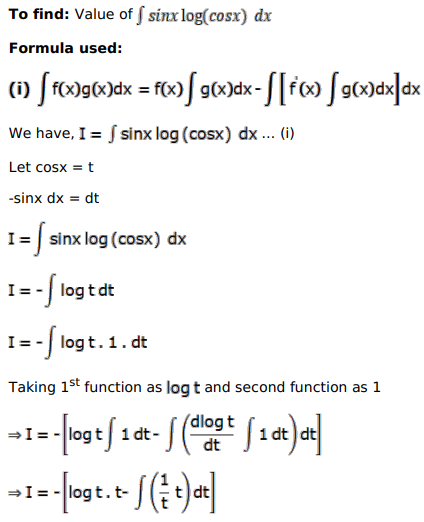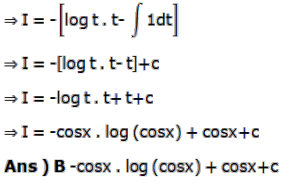# Mark against the correct answer in each of the following:

Question:

Mark $(\sqrt{)}$ against the correct answer in each of the following:

$\int \sin x \log (\cos x) d x=?$

A. $\cos x \log (\cos x)-\cos x+C$

B. $-\cos x \log (\cos x)+\cos x+C$

C. $\cos x \log (\cos x)+\cos x+C$

D. none of these

Solution: